# XMATCH

The XMATCH function returns the position of a value in a list, table or cell range.
This function replaces the MATCH function.
This function defaults to "exact match" instead of "closest match or the next smallest" (which MATCH did).
This function is not case sensitive.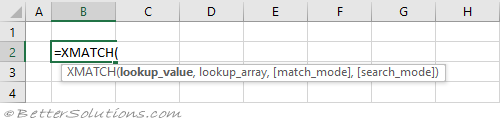### Return the Exact Position (unique values)

This function will return the relative position of the "lookup_value" in the "lookup_array".
When the "match_mode" argument is 0 an exact match is found. This is the default.
The list does not have to be sorted when looking for an exact match.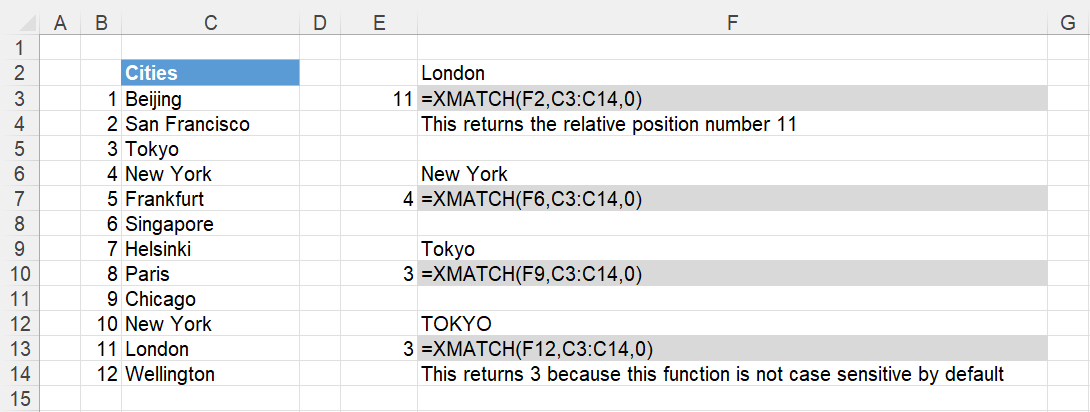### Return the Exact Position (item does not exist)

If the "lookup_value" is not in the list, #N/A is returned.
When the "match_mode" argument is 0 an exact match is found. This is the default.
The list does not have to be sorted when looking for an exact match.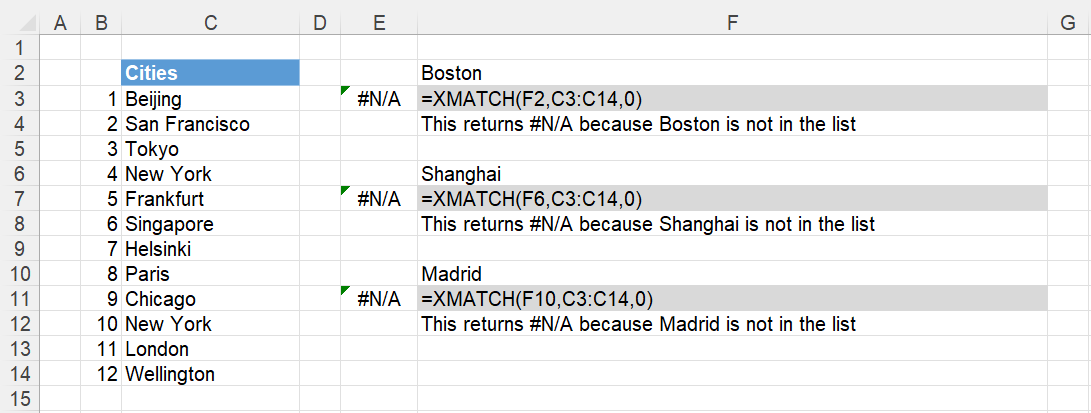### Return the Exact Position (case sensitive, EXACT)

The XMATCH function is not case sensitive.
This means that upper case and lower case characters are equal (for example Tokyo = TOKYO).
You can perform a case sensitive lookup by using the EXACT function.
The list does not have to be sorted when looking for an exact match.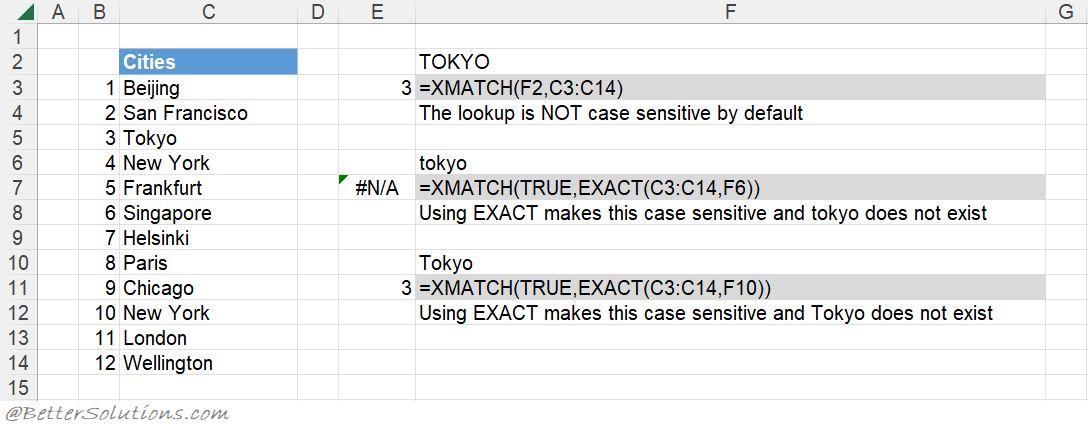### Return the Exact Position (first occurrence, sorted or not sorted)

This function will always return the position of the first occurrence, starting at the top.
When the "match_mode" argument is 0 an exact match is found. This is the default.
The list does not have to be sorted to return the first occurrence.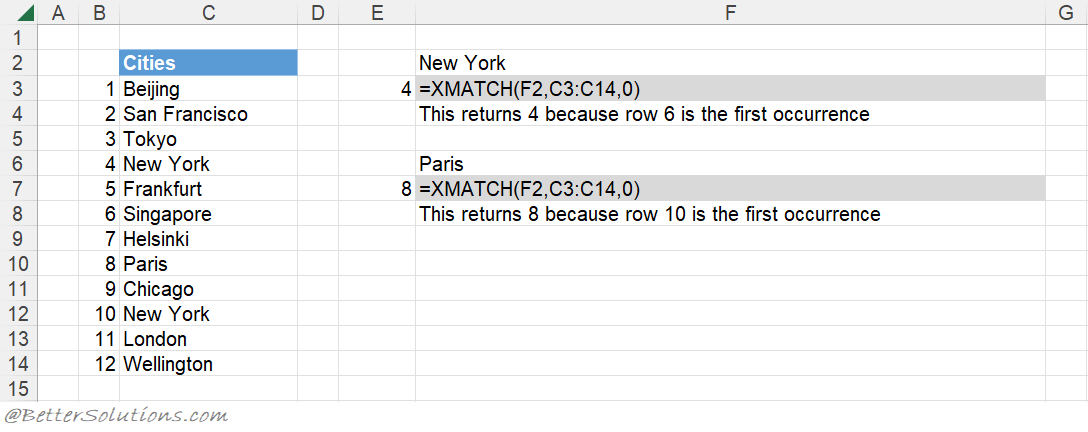### Return the Exact Position (last occurrence, not sorted)

You can get the last occurrence when the list is not sorted by using the MAX, MIN, IF and ROW functions.
The largest value from an array of matching row numbers.
You can swap the MIN function for LARGE or SMALL to return the nth largest or nth smallest positions.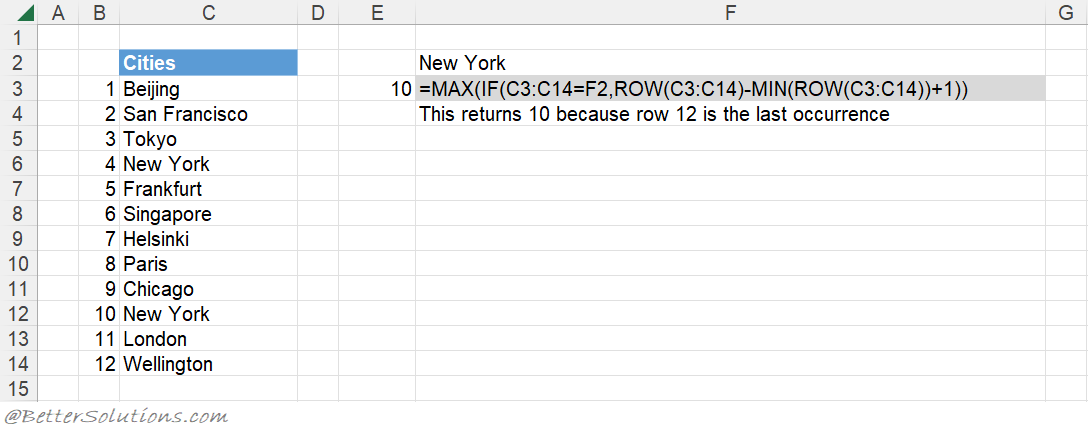### Return the Exact Position (with wildcard *)

An asterisk (*) matches any sequence of text characters (not numbers).
The "match_mode" argument must be 0.
You can have an asterisk as the first character.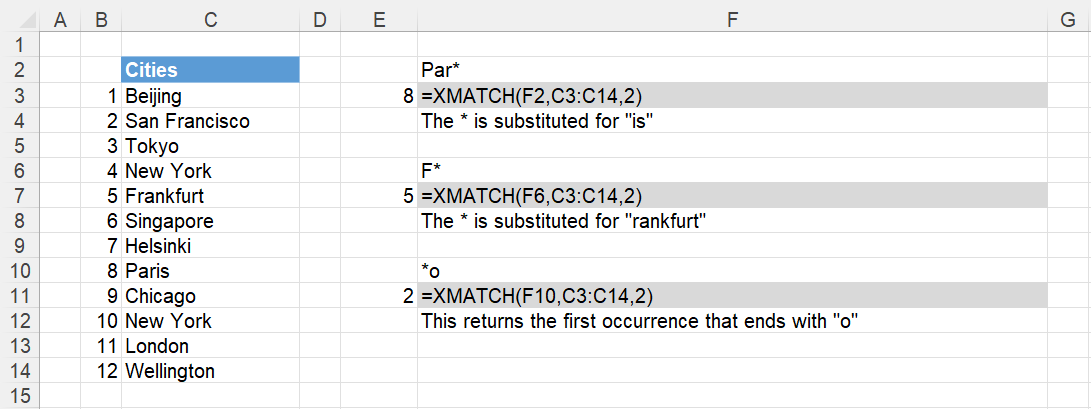### Return the Exact Position (with wildcard ?)

A question mark (?) matches any single text character (not numbers).
The "match_mode" argument must be 0.
You can have a question mark as the first character.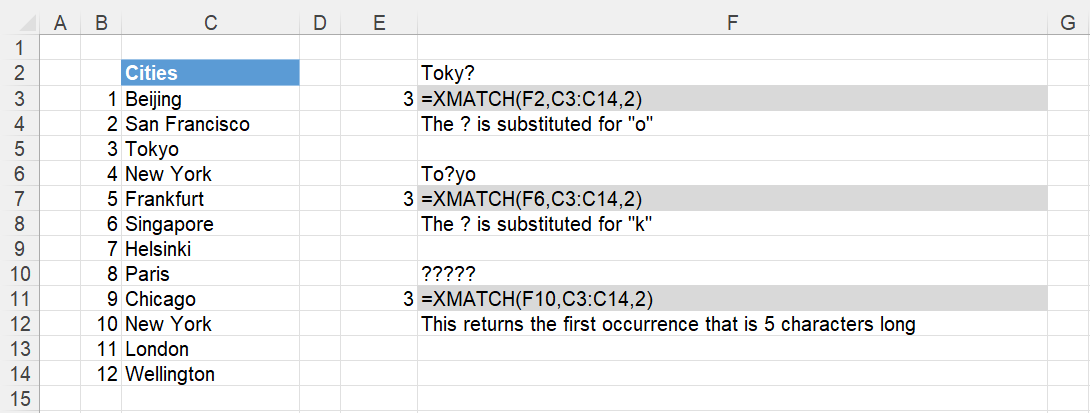### Return the Closest Position (next smallest)

When the "match_mode" argument is 1 the closest value, less than (or equal to) "lookup_value" is returned.
When the "match_mode" argument is 1 the function assumes the list is sorted into Ascending Order.
This list has been sorted into ascending order, smallest first (a-z).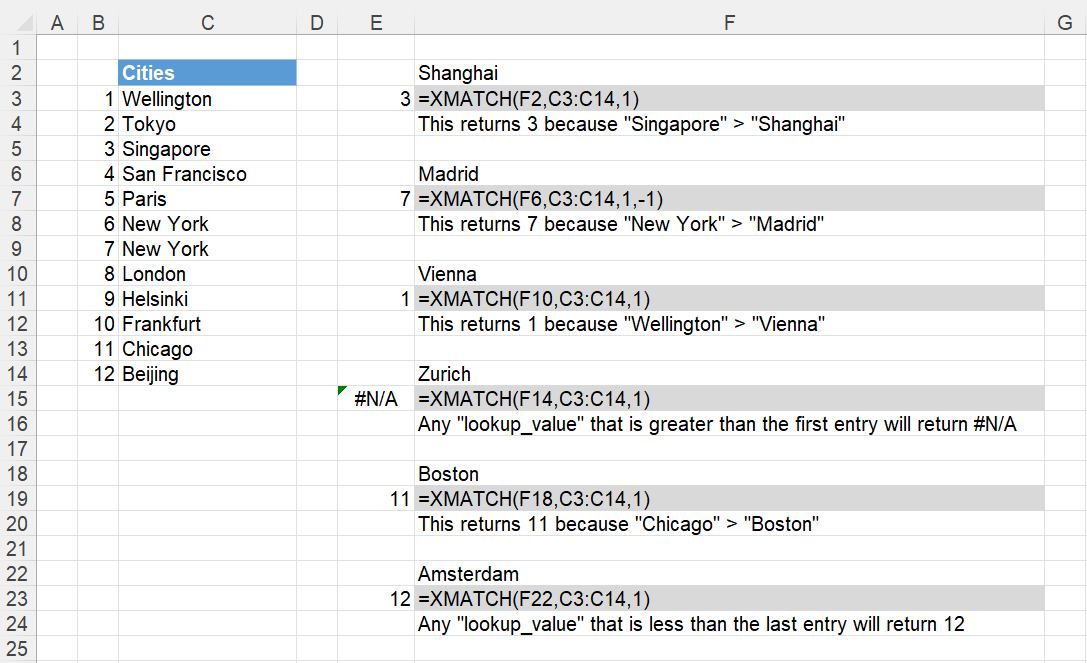### Return the Closest Position (next largest)

When the "match_mode" argument is -1 the closest value, greater than (or equal to) "lookup_value" is returned.
When the "match_mode" argument is -1 the function assumes the list is sorted into Descending Order.
This list has been sorted into descending order, largest first (z-a).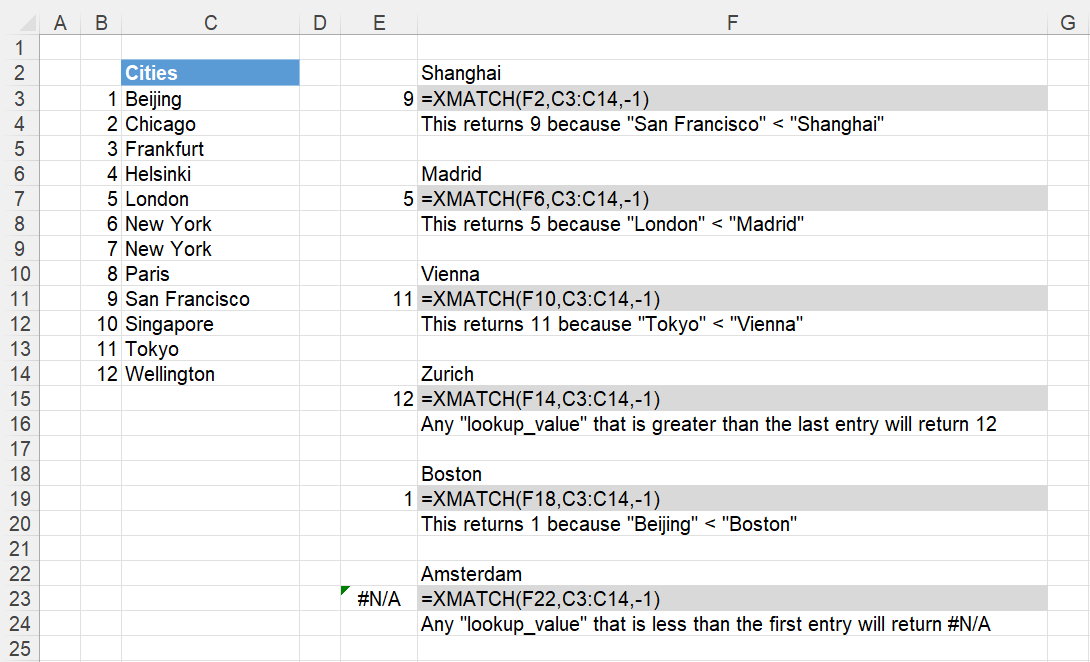### Return the Closest Position (with wildcards)

You cannot have a wildcard character as your first character when the "match_mode" argument is not 0.
If your "lookup_value" begins with an asterisk and the "match_mode" argument is not 0, the "lookup_value" is taken to be a literal string.
If your "lookup_value" begins with a question mark and the "match_mode" argument is not 0, the "lookup_value" is taken to be a literal string.
When the "match_mode" argument is 1 the closest value, less than (or equal to) "lookup_value" is returned.
When the "match_mode" argument is 1 the function assumes the list is sorted into Ascending Order.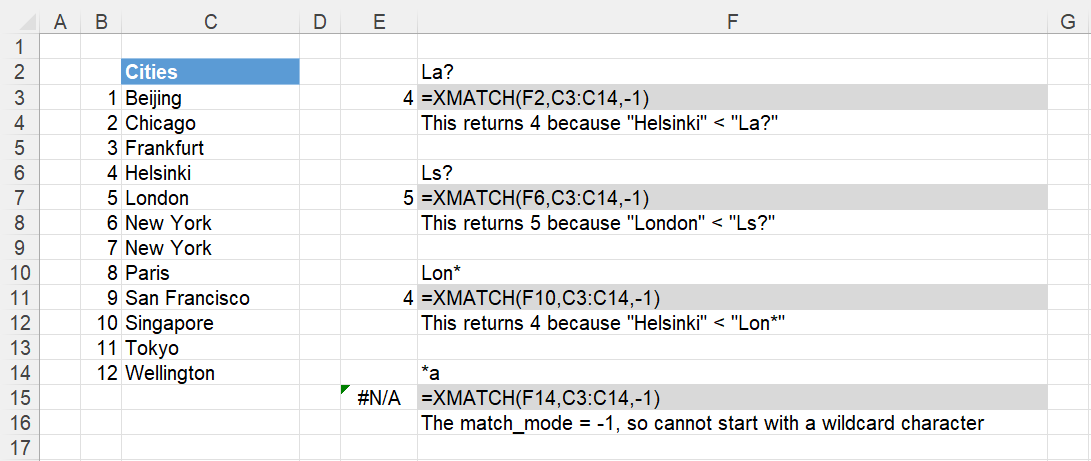### Combining with INDEX

You can use the INDEX and MATCH functions as an alternative to using the VLOOKUP or HLOOKUP functions.